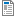National Curriculum

# Year 6 Maths Plans

We provide Hamilton Year 6 maths both as weekly plans (below) and as short blocks. We will eventually be phasing out the plans, as we believe our short blocks offer you all of the same advantages and more. Find out more about the advantages of Hamilton's short blocks.

In order to ensure that every part of the Year 6 content is covered before the statutory assessments in late May, there are nearly 13 weeks of teaching in the Autumn Term, 11 weeks in Spring and 27 days before the SATs in Summer. Each teacher will need to pace this teaching to suit their class and, crucially, their term dates. You may need to consider bringing some of the Spring Term teaching into Autumn. For more support in how to arrange this around different potential term structures structures, read more.

Supporting documents for set
• Week
• Title
1
+ Details

Understand place value in 6-digit numbers. Place 6-digit numbers on a line. Compare 6-digit numbers. Add and subtract 1s, 10s, 100s, 1000s, 10,000s and 100,000s. Use compact column addition to add any pair of 5 digit numbers.Autumn Week 1 Plan
2
+ Details

Understand place value in numbers with 3 decimal places, compare, place on lines and begin to round to the nearest whole, 0.1 or 0.01. Multiply and divide by 10, 100 and 1000 to give answers with 0, 1, 2 or 3 decimal places. Solve problems involving number. Use compact column addition to add 2 or 3 amounts of money or numbers with 2 decimal places in a measures context, e.g. metres, rounding to approximate. Includes bar model examples.Autumn Week 2 Plan
3
+ Details
Subtraction

Add several prices, then find change from £50 and £100 and a difference between two amounts of money using counting up subtraction (Frog). Use compact decomposition to subtract pairs of 5-digit numbers and to subtract 3-digit and 4-digit numbers from 5-digit numbers. Choose whether to use counting up (Frog) or decomposition to subtract pairs of 5-digit numbers. Includes bar model examples.

4
+ Details
Shape and angles

Name parts of circles (radius, diameter, circumference) and know that the diameter is twice the radius. Classify and sort quadrilaterals. Find unknown angles in any triangles, quadrilaterals, and regular polygons. Find unknown angles around a point, on a straight line or vertically opposite.

5
+ Details
Multiplication, division and fractions

Find common multiples and factors. Find numbers that have a pair of prime factors. Find equivalent fractions. Simplify fractions using multiples and factors. Compare and order fractions with unrelated denominators. Find non-unit fractions of numbers using short division and mental multiplication. Includes bar model examples.

6
+ Details
Number and written multiplication

Place 5-digit numbers on a line and round to the nearest 10, 100 or 1000. Place 6-digit numbers on a line and round to the nearest 10, 100, 1000, 10,000 or 100,000. Use short multiplication to multiply 4-digit numbers and 4-digit amounts of money by single-digit numbers. Use rounding to approximate.

7
+ Details
Fractions and division

Recognise fraction and decimal equivalents. Use short division to divide 3-digit and 4-digit numbers by 1-digt numbers and by 11 and 12; round up or down. Write answers with remainders as fractions, e.g. 23¾, simplify fractions and write as decimals, e.g. 23.75.

8
+ Details
Place value, decimals and subtraction

Add/subtract multiples of 0.01 to/from numbers with two decimal places, crossing multiples of 0.1 and 1. Subtract numbers with one or two decimal places by counting up from the smaller to the larger number (Frog), e.g. 3.76 – 1.8 or 13.4 – 2.76. Understand place value in numbers with three decimal places. Count on and back in steps of 0.001 and 0.01 and add and subtract multiples of 0.1, 0.01 or 0.001. Includes bar model examples.

9
+ Details
Measures including time

Convert between grams and kilograms, millilitres and litres (up to 3 decimal places). Convert between metres and kilometres. Know approximate conversions between common imperial units used in daily life and metric units. Draw line graphs and read intermediate points. Read timetables using the 24-hour clock and calculate time intervals and add lengths of times.

10
+ Details
3D shape and fractions

Identify, describe and build 3D shapes using nets. Turn improper fractions into mixed numbers and vice versa. Compare, order, add and subtract fractions with unrelated denominators.

11
+ Details
Written multiplication, mixed calculations and word problems

Use grid multiplication to multiply 3-digit numbers by 2-digit numbers. Use long multiplication to multiply 3-digit numbers by numbers between 10 and 20, then between 20 and 30. Solve a mix of +, –, × and ÷ mental and written calculations. Choose which operations are necessary to solve single-step and multi-step word problems.